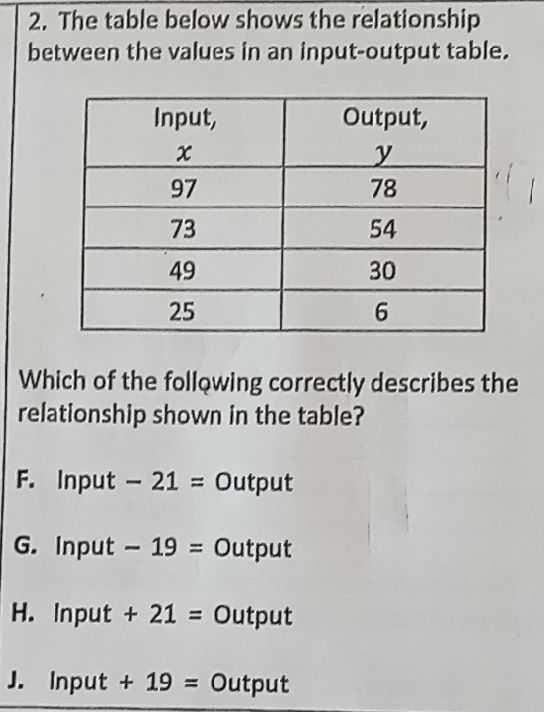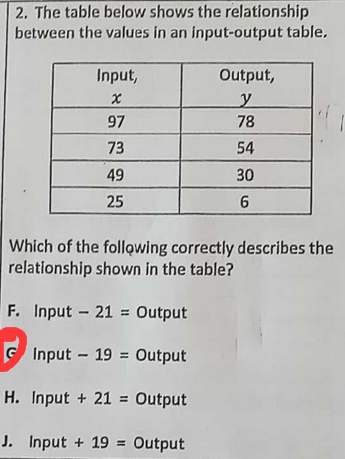### ¿Todavía tienes preguntas de matemáticas?

Pregunte a nuestros tutores expertos
Algebra
Pregunta2. The table below shows the relationship between the values in an input-output table.

Which of the following correctly describes the relationship shown in the table?

F. Input $$- 21 =$$ Output

G. Input $$- 19 =$$ Output

H. Input $$+ 21 =$$ Output

J. Input $$+ 19 =$$ Output